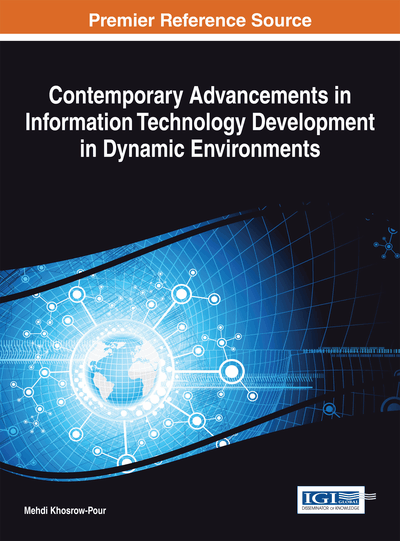# Vague Correlation Coefficient of Interval Vague Sets and its Applications to Topsis in MADM Problems

John Robinson P. (Bishop Heber College (Autonomous), India) and Henry Amirtharaj E. C. (Bishop Heber College (Autonomous), India)
Copyright: © 2014 |Pages: 34
DOI: 10.4018/978-1-4666-6252-0.ch008

## Abstract

Various attempts are made by researchers on the study of vagueness of data through Intuitionistic Fuzzy sets and Vague sets, and also it is shown that Vague sets are Intuitionistic Fuzzy sets. However, there are algebraic and graphical differences between Vague sets and Intuitionistic Fuzzy sets. In this chapter, an attempt is made to define the correlation coefficient of Interval Vague sets lying in the interval [0,1], and a new method for computing the correlation coefficient of interval Vague sets lying in the interval [-1,1] using a-cuts over the vague degrees through statistical confidence intervals is also presented by an example. The new method proposed in this work produces a correlation coefficient in the form of an interval. The proposed method produces a correlation coefficient in the form of an interval from a trapezoidal shaped fuzzy number derived from the vague degrees. This chapter also aims to develop a new method based on the Technique for Order Preference by Similarity to Ideal Solution (TOPSIS) to solve MADM problems for Interval Vague Sets (IVSs). A TOPSIS algorithm is constructed on the basis of the concepts of the relative-closeness coefficient computed from the correlation coefficient of IVSs. This novel method also identifies the positive and negative ideal solutions using the correlation coefficient of IVSs. A numerical illustration explains the proposed algorithms and comparisons are made with some existing methods.
Chapter Preview
Top

## Introduction

Various attempts are made by researchers on the study of data vagueness through intuitionistic fuzzy sets and Vague Sets (VSs). Gau & Buehrer, (1994) introduced the concept of vague sets, and it was shown that vague sets are indeed intuitionistic fuzzy sets (Bustince & Burillo, 1996). But Lu & Ng, (2005, 2009) based their research on the algebraic and graphical differences between vague sets and intuitionistic fuzzy sets. Combining interval-valued fuzzy sets and vague sets, Zhi-feng et al., (2001) introduced the concept of Interval Vague Sets (IVSs). Gau & Buehrer, (1994), Li & Rao, (2001), Liu, P.D., (2009) and Liu, P.D., & Guan, (2008; 2009) have detailed the essential operations of vague sets and interval vague sets. Interval vague set is one of the higher order fuzzy sets and is being applied in various fields. The notion of truth membership function, false membership function and uncertainty function in interval vague sets describes the objective world more realistically and practically. Interval vague sets reflect people’s understanding in three aspects comprehensively: Support degree, Negative degree and Uncertainty degree.

In this work, an attempt is made initially to define the correlation coefficient of interval vague sets lying in the interval [0,1]. An another attempt is made to compute the correlation coefficient of interval vague sets lying in the interval [-1,1] using α-cuts over the vague degrees through statistical confidence intervals, producing a correlation coefficient in the form of an interval. Many of the previously defined correlation coefficient for imprecise data lie in the interval [0,1], except for a very few authors who tried to make it fall in the interval [-1,1]. The correlation coefficient of interval vague sets is defined on the usual interval [0,1] and then a new computational method is proposed which allows the correlation coefficient of interval vague sets to fall in the interval [-1,1]. The novelty of this approach is that the correlation coefficient of interval vague sets is obtained in the form of an interval rather than a single point measure. Statistical confidence intervals and α-cuts are used to construct a kind of trapezoidal fuzzy number derived from the vague degrees.

Of the numerous approaches available for Decision Support Systems (DSS), one most prevalent is the Technique for Order Preference by Similarity to Ideal Solution (TOPSIS), first developed by Hwang & Yoon, (1981). TOPSIS is a logical decision-making approach, dealing with the problem of choosing a solution from a set of candidate alternatives characterized in terms of some attributes. The merit of the TOPSIS method suggested by Hwang & Yoon, (1981) is that it deals with both quantitative and qualitative assessments in the process evaluation with less computation. It is based upon the concept that the chosen alternative should have the shortest distance from the positive ideal solution and the farthest from the negative ideal solution. In the TOPSIS process, the performance ratings and the weights of the criteria are given as crisp values. In fuzzy TOPSIS, attribute values are represented by fuzzy numbers. Janic, (2003) stated that the TOPSIS method embraces seven steps which are:

• 1.

Construction of normalized decision matrix;

• 2.

Construction of weighted-normalized decision matrix;

• 3.

Determining positive ideal and negative ideal solution;

• 4.

Calculating the separation measure of each alternative from the ideal one;

• 5.

Calculating the relative distance of each alternative to the ideal and negative ideal solution;

• 6.

Ranking alternatives in descending order with respect to relative distance to the ideal solution;

• 7.

Identifying the preferable alternative as the closest to the ideal solution.

## Complete Chapter List

Search this Book:
Reset